### Submit a Resource

NRICH: Exploring Cubic Functions

Quadratic graphs are very familiar, but what patterns can you explore with cubics?

In the GeoGebra applet below, move the slider to change the function.
What stays the same? What changes?

Can you take a guess at what function is being graphed each time?
You can check using the Show Equation box.

Here is a second family of functions to explore. In this case, the three roots sum to zero.

What do you notice about the graphs and their functions?
What do they have in common?
Can you explain any of the patterns that you notice?

Here are six graphs and their functions. Can you match the functions to their graphs?

 A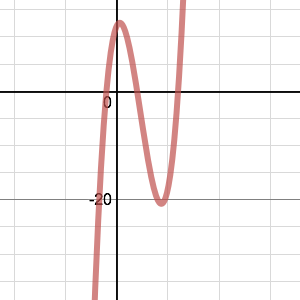B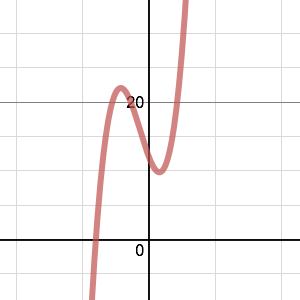C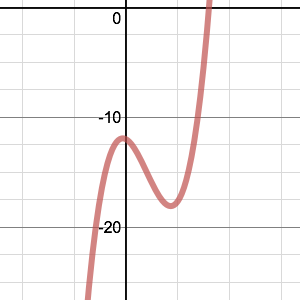D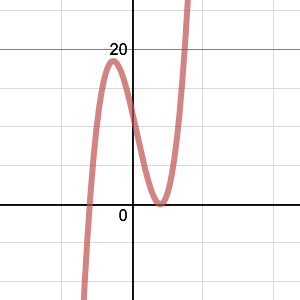E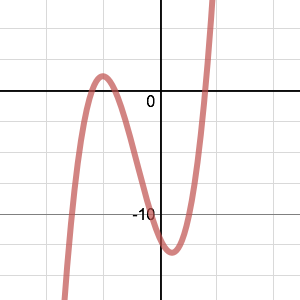F(1)   y=x3+2x25x+12
(2)   y=x3+3x24x12
(3)   y=x37x2+4x+12
(4)   y=x33x2x12
(5)   y=x3+x28x12
(6)   y=x3x28x+12

And finally…

The illustration below shows the graphs of fourteen functions.
Two of them have equations

y=(x+6)32
y=(x9)3+3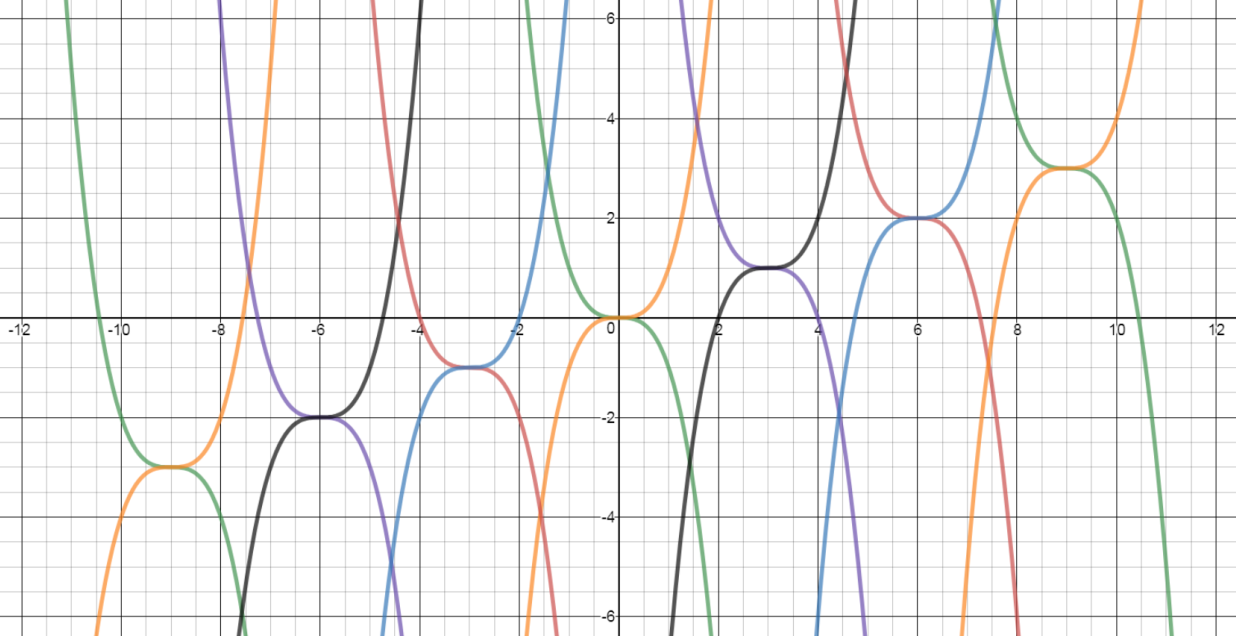Can you find the equations of the other twelve graphs in this pattern?
Can you create some similar patterns of your own, using different families of cubic functions?

With thanks to Don Steward, whose ideas formed the basis of this problem.
Age 14 to 18

Math Topics
Algebra & Pre-Algebra, Exponential Equations, Functions & Graphs
High School, Educator

## Organization

NRICH (University of Cambridge)

## Type of Resource

Challenge
Online Interactive Activity